Disclaimer

All materials and contents are provided for information purposes only. Moscow Exchange assumes no obligations, and makes no representations or warranties, whether express or implied, with regard to the accuracy, completeness, quality, merchantability, correctness, compliance with any specific methodologies and descriptions or the fitness for any particular purpose as well as volume, structure, format, submission dates and timeliness, of such materials and contents. Any such materials and contents (or any portion thereof) cannot be used for any investment or commercial purposes including the creation of financial instruments, products or indices.

Aggregated Netflow analytics 2¶

Aggregated statistics on clients with the highest netflow¶

Content:¶

• Brief product description
• Methodology
• Fields description
• Data review
• Creating derived features
• Direction of netflow and tomorrow price movement
• Resume
• Contacts

Brief product description¶

The product includes aggregated daily netflow (buy volume - sell volume) of top 30, 70 and 100 clients by absolute value of netflow in number of shares and RUB. All trades of all clients in order driven trading mode starting from 10:00 A.M. till to 6:30 P.M. are taken into account for calculations. At 6:30 P.M. the clients with a highest absolute value of netflow are selected and their netflows are summarized. At the end of the day there are three values for top 30, 70 and 100 clients in number of shares and in RUB.

Currently netflow values available for the following 10 equities: SBER, GAZP, LKOH, GMKN, VTBR, ROSN, MGNT, ALRS, SBERP, AFLT

Methodology¶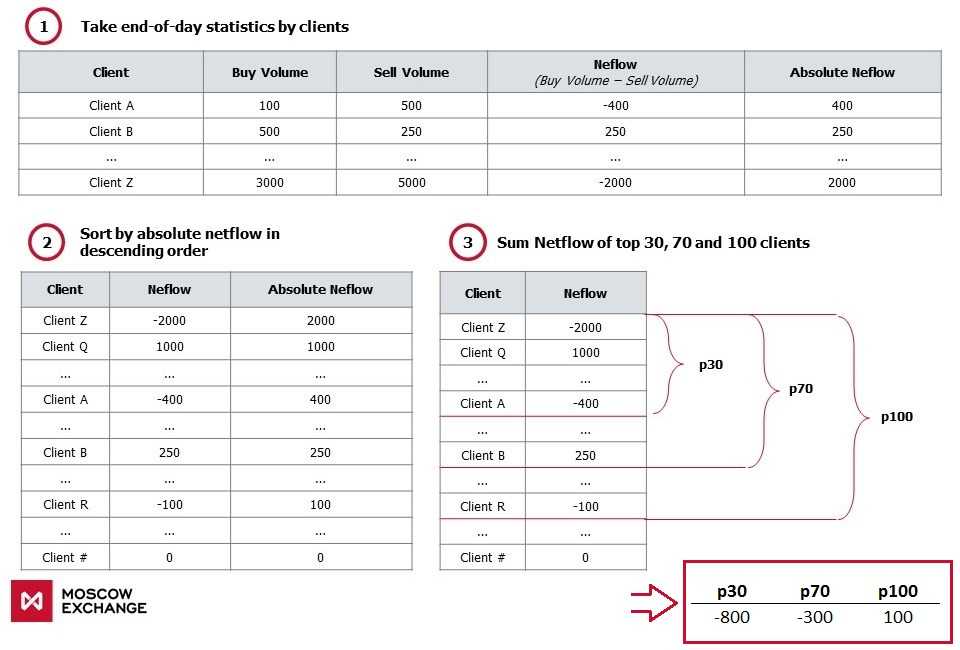Fields description¶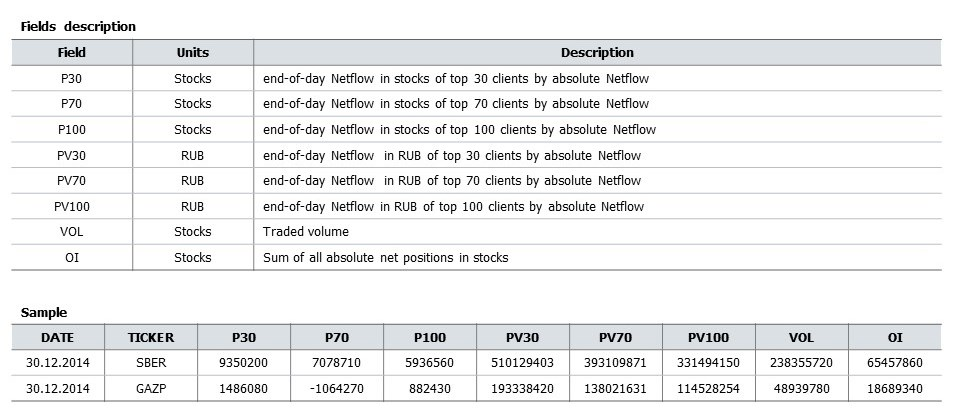Key defenitions used below:¶

1. p30, p70, p100 - Aggregated netflow by 30, 70 and 100 clients, in shares
2. today_change - today's price change (today's close to open), in %
3. tomorrow_change - tomorrow's price change (tomorrow's close to today's close), in %

Data review¶

In :
import pandas as pd
import numpy as np
import matplotlib.pyplot as plt
%pylab inline
Populating the interactive namespace from numpy and matplotlib
In :
plt.rcParams['figure.figsize'] = (15, 8)
plt.style.use('ggplot')

Let's consider data on SBER equity (Sberbank of Russia). We will read historical values of netflow for 4 years (2014-2017)

In :
ticker = 'SBER'
In :
X = pd.read_csv('../netflows_2014_2017/' + ticker + '_netflow.csv')
X['date'] = pd.to_datetime(X['date'])
X = X.set_index('date')
X = X[['p30', 'p70', 'p100', 'oi']][3:]
Out:
p30 p70 p100 oi
date
2014-01-10 3333680 1310760 1126430 31124820
2014-01-13 4419280 3384600 2836260 39212280
2014-01-14 -3718740 -3441470 -2529540 37688760
2014-01-15 8258230 6357870 5086300 46134300
2014-01-16 2398870 2589590 2222780 41385660
In :
# Key statistics
X.describe()
Out:
p30 p70 p100 oi
count 1.000000e+03 1.000000e+03 1.000000e+03 1.000000e+03
mean 1.979914e+05 1.056551e+05 8.627090e+04 6.095437e+07
std 7.998605e+06 6.286671e+06 5.414825e+06 3.578948e+07
min -3.759355e+07 -3.086781e+07 -2.594362e+07 8.448360e+06
25% -3.560330e+06 -2.634188e+06 -2.219885e+06 3.513840e+07
50% -6.378500e+04 -9.621000e+04 -1.111850e+05 5.310237e+07
75% 3.366888e+06 2.579210e+06 2.074978e+06 7.751528e+07
max 3.208886e+07 2.828582e+07 2.494898e+07 2.902350e+08
In :
# Time-Series chart. Cumulative amount of p30, p70 and p100
X[['p30', 'p70', 'p100']].cumsum().rolling(5).mean().plot()
Out:
<matplotlib.axes._subplots.AxesSubplot at 0x1963604f438>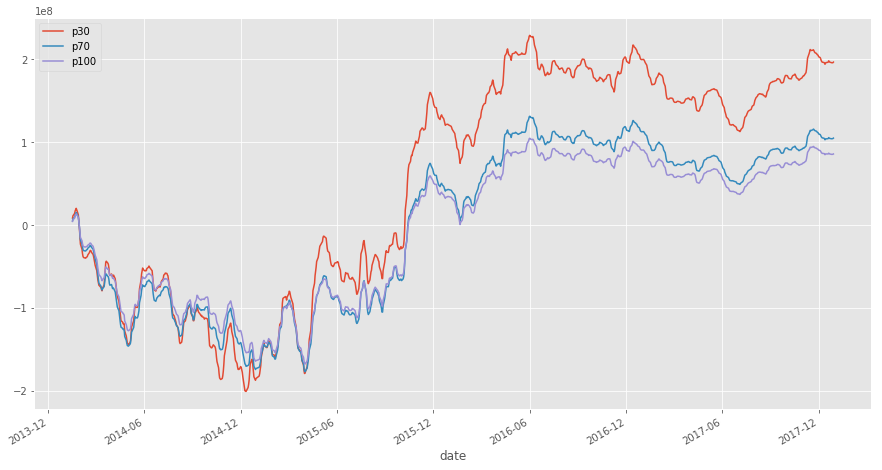In :
# Distribution of values p30, p70 and p100. Average and median values ~0
X[['p30', 'p70', 'p100']].plot.box()
Out:
<matplotlib.axes._subplots.AxesSubplot at 0x19636383748>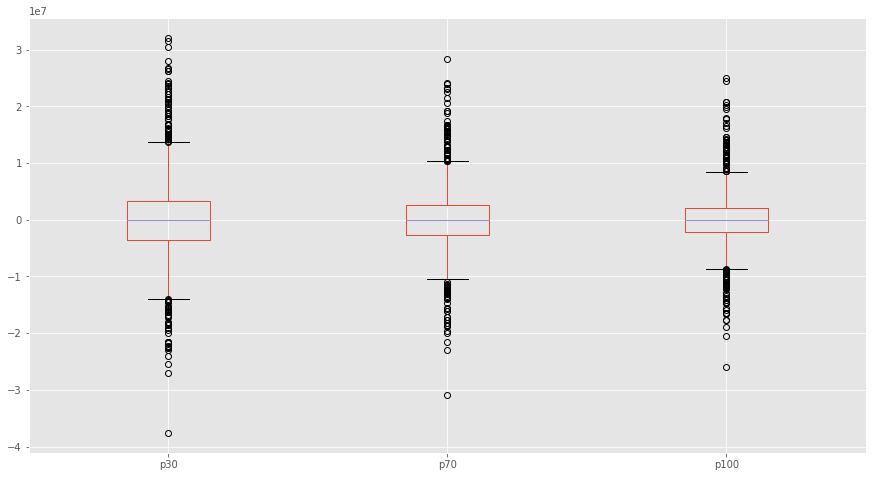Let's calculate the correlations between p30, p70, p100 and today_change, tomorrow_change. Downloading historical prices and volumes - OHLCV:

In :
# function to get OHLCV daily values for a given ticker and for a given time period using MOEX API
def get_ohlcv(ticker, date_from, date_till):
P = pd.DataFrame()

# using 'for' loop because max outputs are limited by 500 rows
for i in range(5):
url = 'http://iss.moex.com/iss/engines/stock/markets/shares/boards/tqbr/securities/' + ticker + '/candles.csv' \
'?from=' + date_from + \
'&till=' + date_till + \
'&interval=24' \
'&start=' + str(500*i)
P = P.append(pd.read_csv(url, ';',skiprows=2))
P['date'] = P.apply(lambda row: row['begin'][:10], axis =1)
P['date'] = pd.to_datetime(P['date'], format = '%Y-%m-%d')
P = P.set_index('date')

#subtracting by 2, to get Turnover from Volume
P['volume'] = 2 * P['volume']
P['value'] = 2 * P['value']

P.drop(['begin', 'end'], axis = 1, inplace = True)
return P
In :
Y = get_ohlcv(ticker, '2014-01-01', '2018-01-30')
In :
# change - today's price change (close to open)
Y['today_change'] = 100 * (Y['close'] - Y['open']) / Y['open']

# tom_change - tomorrow's price change (close(+1) to сlose(0))
Y['tomorrow_change'] = 100 * Y['close'].pct_change().shift(-1)

Out:
open close high low value volume today_change tomorrow_change
date
2014-01-06 100.20 98.91 100.31 98.62 6.308941e+09 63383600 -1.287425 -0.727934
2014-01-08 99.10 98.19 99.41 97.85 8.359877e+09 84744580 -0.918264 -0.193502
2014-01-09 98.44 98.00 98.77 97.69 9.036778e+09 91973800 -0.446973 1.224490
2014-01-10 97.87 99.20 99.41 97.52 1.021936e+10 103804800 1.358946 1.058468
2014-01-13 99.30 100.25 100.35 99.04 1.238301e+10 124102500 0.956697 -1.007481
In :
# Adding price changes to the netflow data frame
X = pd.merge(X, Y[['today_change', 'tomorrow_change', 'volume', 'value']], how='left', left_index = True, right_index = True)
Out:
p30 p70 p100 oi today_change tomorrow_change volume value
date
2014-01-10 3333680 1310760 1126430 31124820 1.358946 1.058468 103804800 1.021936e+10
2014-01-13 4419280 3384600 2836260 39212280 0.956697 -1.007481 124102500 1.238301e+10
2014-01-14 -3718740 -3441470 -2529540 37688760 0.060496 1.541717 156970320 1.556252e+10
2014-01-15 8258230 6357870 5086300 46134300 0.820410 -0.119083 155678740 1.561511e+10
2014-01-16 2398870 2589590 2222780 41385660 -0.247770 0.516642 125518560 1.268416e+10
In :
# Time-Series chart. Cumulative amount of p30, p70, p100 and today's price change - today_change (black line)
X[['p30', 'p70', 'p100']].cumsum().rolling(5).mean().plot()
plt.ylabel('Number of shares')
X['today_change'].cumsum().rolling(5).mean().plot(secondary_y = True, c='#000000')
plt.ylabel('%')
Out:
Text(0,0.5,'%')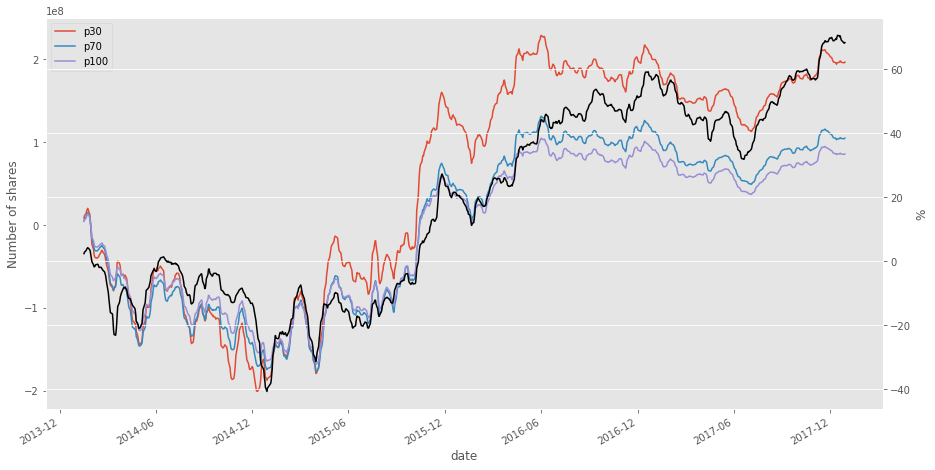Correlation between p30, p70, p100 and today_change, tommorow_change for 4 years

In :
X[['p30', 'p70', 'p100', 'today_change', 'tomorrow_change']].corr()
Out:
p30 p70 p100 today_change tomorrow_change
p30 1.000000 0.983902 0.978002 0.739199 0.085658
p70 0.983902 1.000000 0.995490 0.746328 0.094855
p100 0.978002 0.995490 1.000000 0.753358 0.088971
today_change 0.739199 0.746328 0.753358 1.000000 0.070943
tomorrow_change 0.085658 0.094855 0.088971 0.070943 1.000000
• Strong correlation between p30, p70 and p100 (~0.98)
• Strong positive correlation between p30, p70, p100 and today_change (~0.75)
• Weak positive correlation between p30, p70, p100 and tomorrow_change (~0.09)

Therefore, it can be assumed that today_change is connected with p30, p70 and p100. Also, there is weak stable connection between netflow and tommorow_change.

Maybe it is possible to increase correlation between p30, p70, p100 and tommorow_change:

1. Let's normalize p30, p70 и p100 on trading volume - VOLUME (OI, log(VOLUME) ...)
2. Calculate p30, p70 и p100 changes regarding previous N days.

Creating derived features¶

In :
Z = X.copy()
Z['p30_vol'] = 100 * Z['p30'] / Z['volume']
Z['p70_vol'] = 100 * Z['p70'] / Z['volume']
Z['p100_vol'] = 100 * Z['p100'] / Z['volume']
Z = Z.astype(float)
In :
Z.corr()
Out:
p30 p70 p100 oi today_change tomorrow_change volume value p30_vol p70_vol p100_vol
p30 1.000000 0.983902 0.978002 0.116485 0.739199 0.085658 0.112916 0.136930 0.879253 0.879285 0.882764
p70 0.983902 1.000000 0.995490 0.093715 0.746328 0.094855 0.088105 0.110940 0.854245 0.884921 0.890116
p100 0.978002 0.995490 1.000000 0.094082 0.753358 0.088971 0.086250 0.111846 0.841797 0.873696 0.887359
oi 0.116485 0.093715 0.094082 1.000000 0.061604 0.088350 0.932113 0.784842 0.063978 0.058432 0.061230
today_change 0.739199 0.746328 0.753358 0.061604 1.000000 0.070943 0.064108 0.121885 0.685178 0.700282 0.712124
tomorrow_change 0.085658 0.094855 0.088971 0.088350 0.070943 1.000000 0.080983 0.082697 0.100420 0.104861 0.102002
volume 0.112916 0.088105 0.086250 0.932113 0.064108 0.080983 1.000000 0.813131 0.058125 0.050007 0.050755
value 0.136930 0.110940 0.111846 0.784842 0.121885 0.082697 0.813131 1.000000 0.083652 0.075511 0.079297
p30_vol 0.879253 0.854245 0.841797 0.063978 0.685178 0.100420 0.058125 0.083652 1.000000 0.979742 0.972260
p70_vol 0.879285 0.884921 0.873696 0.058432 0.700282 0.104861 0.050007 0.075511 0.979742 1.000000 0.994428
p100_vol 0.882764 0.890116 0.887359 0.061230 0.712124 0.102002 0.050755 0.079297 0.972260 0.994428 1.000000

When p30, p70, p100 are normalized on volume, correlation between p30_vol, p70_vol, p100_vol and tomorrow_change became ~0.1

Let's calculate difference(delta) of p30, p70 and p100 regarding their average for previous 1-5 days

In :
cols = ['p30', 'p70', 'p100', 'p30_vol', 'p70_vol', 'p100_vol']

for col in cols:
for i in [1,2,3,4,5]:
Z[col + '_' + str(i)] = Z[col] - Z[col].shift(periods=1, freq=None, axis=0).rolling(i, min_periods = 1).mean()

print('New table shape ' + str(Z.shape))
New table shape (1000, 41)
Out:
p30 p70 p100 oi today_change tomorrow_change volume value p30_vol p70_vol ... p70_vol_1 p70_vol_2 p70_vol_3 p70_vol_4 p70_vol_5 p100_vol_1 p100_vol_2 p100_vol_3 p100_vol_4 p100_vol_5
date
2014-01-10 3333680.0 1310760.0 1126430.0 31124820.0 1.358946 1.058468 103804800.0 1.021936e+10 3.211489 1.262716 ... NaN NaN NaN NaN NaN NaN NaN NaN NaN NaN
2014-01-13 4419280.0 3384600.0 2836260.0 39212280.0 0.956697 -1.007481 124102500.0 1.238301e+10 3.560992 2.727262 ... 1.464546 1.464546 1.464546 1.464546 1.464546 1.200275 1.200275 1.200275 1.200275 1.200275
2014-01-14 -3718740.0 -3441470.0 -2529540.0 37688760.0 0.060496 1.541717 156970320.0 1.556252e+10 -2.369072 -2.192434 ... -4.919695 -4.187423 -4.187423 -4.187423 -4.187423 -3.896894 -3.296757 -3.296757 -3.296757 -3.296757
2014-01-15 8258230.0 6357870.0 5086300.0 46134300.0 0.820410 -0.119083 155678740.0 1.561511e+10 5.304661 4.083968 ... 6.276402 3.816554 3.484787 3.484787 3.484787 4.878654 2.930207 2.680816 2.680816 2.680816
2014-01-16 2398870.0 2589590.0 2222780.0 41385660.0 -0.247770 0.516642 125518560.0 1.268416e+10 1.911168 2.063113 ... -2.020855 1.117346 0.523514 0.592735 0.592735 -1.496299 0.943027 0.457172 0.514313 0.514313

5 rows × 41 columns

In :
# top 10 most correlated features
cols = Z.corr()['tomorrow_change'].sort_values(ascending = False)[1:11]

cols
Out:
p70_3         0.109256
p70_vol_3     0.108843
p70_vol_4     0.107206
p100_vol_3    0.106920
p70_vol       0.104861
p100_vol_4    0.104418
p100_3        0.103789
p70_1         0.102869
p70_vol_5     0.102313
p100_vol      0.102002
Name: tomorrow_change, dtype: float64

Differences of p30, p70 and p100 shows correlation of ~0.11. Correlations on other instruments are in the table below.

TICKERPOS vs TOD_CHGPOS vs TOM_CHGPOS/VOL vs TOM_CHGΔPOS/VOL vs TOM_CHG
SBER0.750.090.100.11
GAZP0.730.090.080.11
LKOH0.650.080.080.13
GMKN0.600.040.070.05
MGNT0.600.040.070.06
ROSN0.670.100.110.12
VTBR0.560.100.010.06
ALRS0.450.000.040.07
SBERP0.500.070.100.09
AFLT0.500.060.070.07
• POS vs TOD_CHG - correlation between netflow and today_change
• POS vs TOM_CHG - correlation between netflow and tomorrow_change
• POS/VOL vs TOM_CHG - correlation between netflow (divided on volume) and tomorrow_change
• ΔPOS/VOL vs TOM_CHG - correlation between netflow differences (deltas) over previous days and tomorrow_change

One can examine correlation over 4 years.

Сo-directionality of netflow and tommorow_change statistics¶

Averages of p30, p70 and p100 and their differencies are located near zero. Let's calculate co-directionality statistics of netflow and tommorow_change in days and percentages based on p70_vol_3 (correlation with tommorow-change ~ 0.11):

*difference between p70 normalized on volume and its average over the 3 previous days

In :
Z = Z[1:]
Z = Z[Z['tomorrow_change'] != 0]
Z['feature'] = np.sign(Z['p70_vol_3'])
Z['base'] = np.sign(Z['tomorrow_change'])
pd.crosstab(index = Z['feature'].astype(int), columns = Z['base'].astype(int))
Out:
base -1 1
feature
-1 248 243
1 244 259

• 248 - Number of days when netflow and tommorow_change are negative (co-directional)
• 259 - Number of days when netflow and tommorow_change are positive (co-directional)
• 243 - Number of days when netflow is negative and tomorrow_change is positive (oppositely directed)
• 245 - Number of days when netflow is positive and tomorrow_change is negative (oppositely directed)
Therefore (248 + 259) = 507 days from 995 when netflow was co-directional with tomorrow_change

In the same way, let's calculate cumulative sum of price percentage changes in case of co-directionality and opposite directionality:

In :
pd.crosstab(index = Z['feature'].astype(int), columns = Z['base'].astype(int), values = Z['tomorrow_change'].abs().astype(int), aggfunc = 'sum')
Out:
base -1 1
feature
-1 280 234
1 190 325
• 280 - Netflow and tomorrow_change are negative
• 325 - Netflow and tomorrow_change are positive
• 234 - Netflow is negative and tomorrow_change is positive
• 191 - Netflow is positive and tomorrow_change is negative

When netflow and tomorrow_change are co-directed, price change sum = 615 (280+325), when opposite directed = 425 (234+191)

Co-directionality statistics based on p70_vol_3 equals 180 (615-425).

* For simplicity, percentages are calculated cumulatively. Example: If price changes are +2%, -1%, +1%, we get +2%

Daily time-series plot of these statistics (black line - SBER price, five colored lines - cumulative co-directionality statistics for top 5 features with the biggest correaltion):

In :
R = pd.DataFrame()
for col in cols.index[:5]:
R[col] = Z.apply(lambda x: x['tomorrow_change'] if x[col] > 0 else (-1 * x['tomorrow_change']), axis=1)
R['base'] = Z['tomorrow_change']
R.cumsum().plot(style=['#CE1126', '#E26EB2', '#FFA100', '#8D3C1E', '#63B1E5', '#000000'])
plt.ylabel('%')
Out:
Text(0,0.5,'%')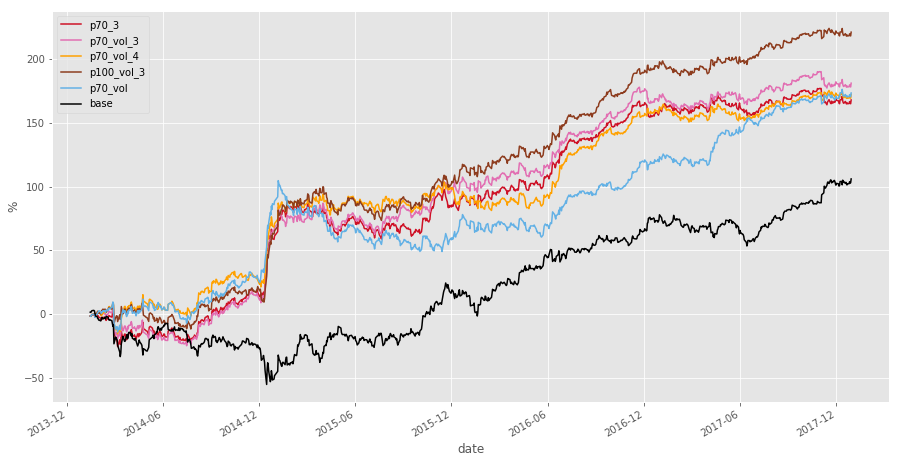Same plots for other instruments: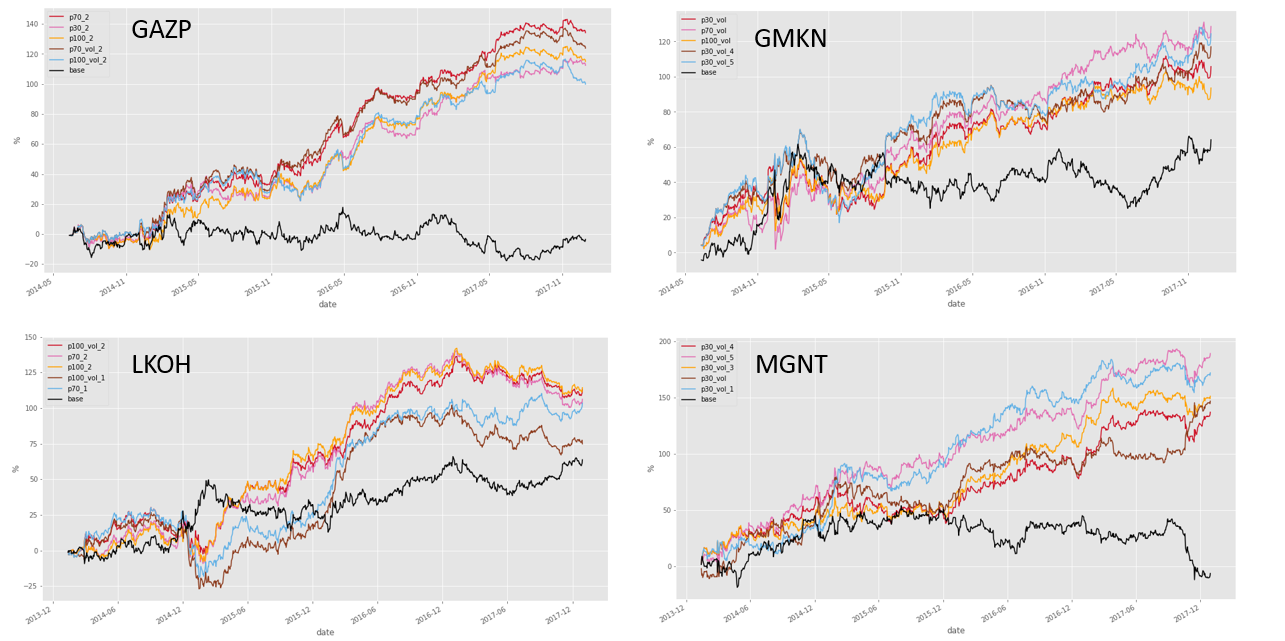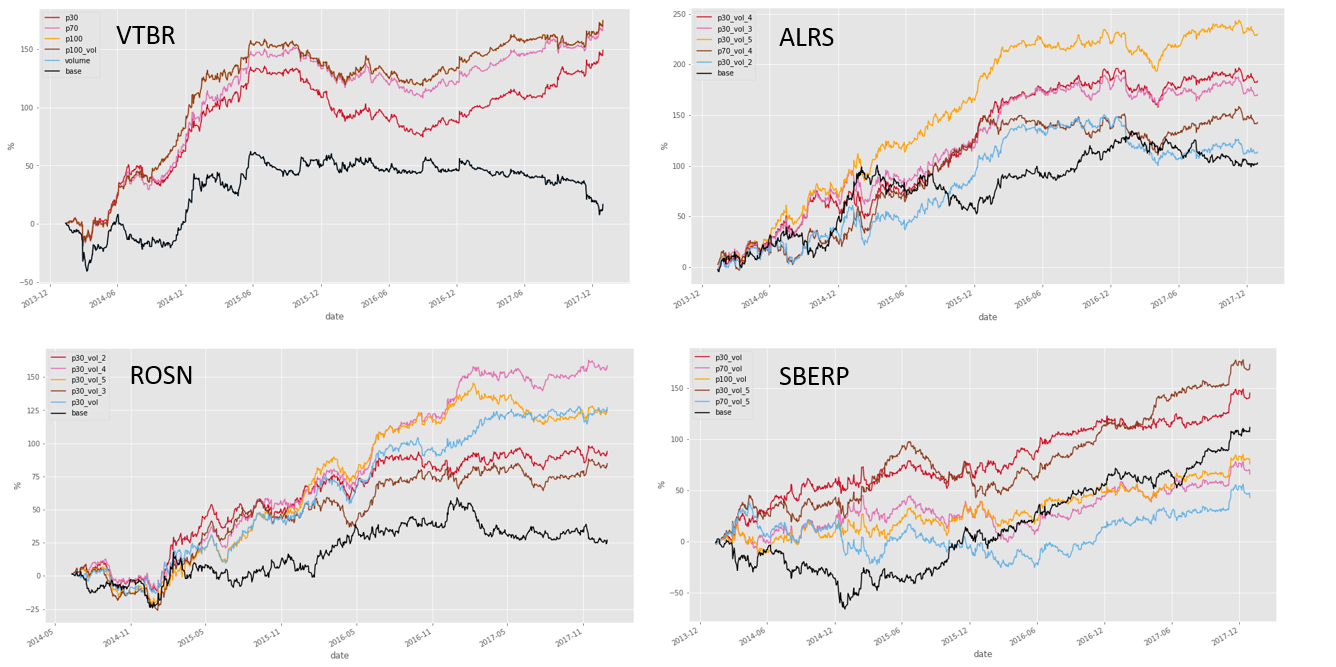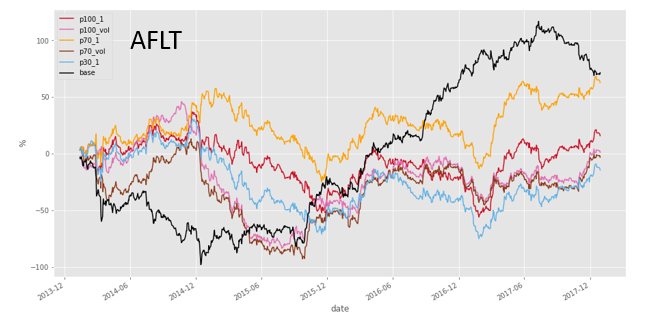Resume¶

1. Using the example of SBER, we calculated the basic statistics, distribution and dynamics of netflow (p30, p70, p100) of large traders in the 2014-2017 period.
2. There is strong correlation between net volume and today_change (~ 0.75) and weak correlation with tomorrow_change, but positive and stable (~ 0.1)
3. The statistics of one of the indicators of netflow (p70_vol_3) with tomorrow_change (correlation ~ 0.11) is calculated: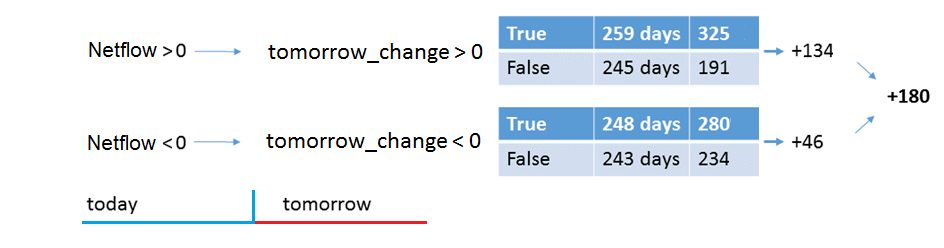Contacts¶

Website - https://moex.com/en/analyticalproducts?netflow2
Email - support.dataproducts@moex.com